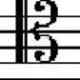# When will the velocity of a particle be perpendicular to it's initial velocity?

2,429

Actually @Ocelo7 has already answered it; I am just showing that in a concise way:

Velocity can be found by differentiating the position-vector:$$\dot{\mathbf{r}}= \mathbf{v}(t) = 10\mathtt{\hat{ i}} + (20 - 10t)\mathtt{\hat{j}}$$ .

Assuming the initial time is $t_0$ & the required time is $t$, we shall use the property $$\mathbf{v}_{t_0}\cdot \mathbf{v_\perp}_{t} = 0 ^1\\\\\\\ \implies 10\cdot 10 \mathtt{\hat{i}}\cdot \mathtt{\hat{i}} + \{\ldots\}\mathtt{\hat {i}}\cdot \mathtt{\hat{j}} + (20 - t_0)\cdot (20-t)\mathtt{\hat{j}}\cdot\mathtt{\hat{j}} = 0 \implies (20-t_0)t=100 + 400 -20t_0$$ . Now, only need to know what $t_0$ is.

$^1$I am assuming you know the relation, but if you don't then here is the little proof:

$\mathbf{A}\cdot \mathbf{B} = AB\cos\theta \implies \mathbf{A}\cdot \mathbf{B_{\perp A}} = AB \cos\left(\frac{\pi}{2}\right) = 0$

Share:
2,429Author by

### Helix

Updated on May 17, 2020

•Helix over 2 years

I am learning kinematics with vector analysis. I was given the position equation:$\mathbf{r} = 10t\hat{\mathtt{i}} + (20t-5t^2)\hat{\mathtt{j}}$. It asks me the time when the velocity of the particle will be perpendicular to its initial velocity. The teacher taught us how to solve it, but I didn't get the concept. Can someone explain the concept? I remembered him solving this problem by using either dot product or cross product of vector. I expect the explanation will not be too difficult for high school student.

•Ryan Unger over 7 years
Hint: you are looking for the condition $\mathbb{v}_i\cdot \mathbb{v}_f=0$, where $\cdot$ is the dot product.
•Carl Witthoft over 7 years
Hi. Please follow the posting guidelines,to wit: post the actual problem in question and what you've done to attempt to solve it.
•Helix over 7 years
Hi thank you. Well Carl actually when posting this question I bear in mind not to ask "please do my homework" question. So I wanted someone to explain the concept. Just like 0celo7 had posted, I wanted to know why we use . Product instead of cross and so on. i'll edit the post.
•Initial means $t=0$?
•Helix over 7 years
Thank you for answering the question, incase the cos tetha is not 0, how can we calculate it ? I meant the AB in the right side of the equation. Just because cos tetha is 0 then it's easier, how about if it's not 0. Thanks
•@HelixTicks; In that case, you need some additional info.
•Helix over 7 years
can you give me a reference on that ? A web site is preferable. I love this topic and hated missing some pieces of information about it
•Okay, can you specify what you want to know? Which stuff've you missed?? I may help if you provide details of your query:)
•Helix over 7 years
The things I missed but trying to comprehend :
•What .............??
•Helix over 7 years
1.So if we want the scalar result of the product of 2 vectors, we use the dot product ? 2. Else, if we want the result in vector form, we use cross product ? 3. Then if the angle between the 2 vectors is not 90 degree, how do we calculate the dot product ? For instance cos 45 is not 0. I mean I know how to solve the left side of the equation but no the right side. Just because cos 90 is 0 then we just put 0. How about the 45 degree thing ? Thank you. Sorry for making 2 comments, intented to make new line.
•See, for these sort of problems, there will be some additional information provided to you; I cannot remember a good numerical problem, but if you start solving problems which many good books do provide, then you'll conceive what I'm talking of; you just need to face the problems.
•You aren't understanding the use of cross product; if you want to know the answers of your query, you have to do many numerical-problems. Each question provides a possible(specific) scenario; how you use them simply depends on you. Why have I used dot product?? Because I know any vector $\perp$ to another one has their dot product $0$; why not cross-product?? Because it's simply irrelevent & worthless to use it here; you know the two vectors & $\sin 90^\circ$ & hence the cross-product. So, what? How could you use it here? Along what thing would you equate the cross-product?? You could use it..
•..if some additional info were given like the value of the cross-product. So, it is better to grab a good book & start numericals; they will enrich you with new facts. BTW, I advise you to first strengthen your math-knowledge like differential & integral calculus; transformations of graphs, series & convergence etc; they will help you in facing the numericals at ease:)
•Helix over 7 years
Thank you user for guiding this problem. Hmm, I suggest you get a more humanlike name. I mean it's awkward to use such robotic name. Here is the problem I faced, I like to learning everything totally. I mean understand it. But the education here are quite broken. I was taught vectors when I've not learnt any trigonometry. And for differential and integral, I was just given the differential and integral of this is this, I learnt nothing about "why". So this is just to give you some image why I'm like a screwed up student. Any good book would you suggest for me to learn from beginning ?
•••••